# The OPTMILP Procedure

## Overview: OPTMILP Procedure

The OPTMILP procedure is a solver for general mixed integer linear programs (MILPs).

A standard mixed integer linear program has the formulation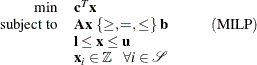where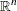is the vector of structural variables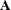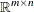is the matrix of technological coefficientsis the vector of objective function coefficients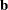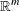is the vector of constraints right-hand sides (RHS)is the vector of lower bounds on variablesis the vector of upper bounds on variables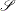is a nonempty subset of the set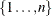of indices

The OPTMILP procedure implements a linear-programming-based branch-and-bound algorithm. This divide-and-conquer approach attempts to solve the original problem by solving linear programming relaxations of a sequence of smaller subproblems. The OPTMILP procedure also implements advanced techniques such as presolving, generating cutting planes, and applying primal heuristics to improve the efficiency of the overall algorithm.

The OPTMILP procedure requires a mixed integer linear program to be specified using a SAS data set that adheres to the mathematical programming system (MPS) format, a widely accepted format in the optimization community. Chapter 15 discusses the MPS format in detail. It is also possible to input an incumbent solution in MPS format; see the section Warm Start for details.

You can use the MPSOUT= option to convert data sets that are formatted for the LP procedure into MPS-format SAS data sets. The option is available in the LP, INTPOINT, and NETFLOW procedures. For details about this option, see Chapter 5: The LP Procedure in SAS/OR 12.3 User's Guide: Mathematical Programming Legacy Procedures, Chapter 4: The INTPOINT Procedure in SAS/OR 12.3 User's Guide: Mathematical Programming Legacy Procedures, and Chapter 6: The NETFLOW Procedure in SAS/OR 12.3 User's Guide: Mathematical Programming Legacy Procedures.

The OPTMILP procedure provides various control options and solution strategies. In particular, you can enable, disable, or set levels for the advanced techniques previously mentioned.

The OPTMILP procedure outputs an optimal solution or the best feasible solution found, if any, in SAS data sets. This enables you to generate solution reports and perform additional analyses by using SAS software.